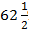# If x% of y is equal to z, what percent of z is x

1.  y2/100

2.  y/1002

3.  100/y

4.  1002/y

4

1002/y

Explanation :
No Explanation available for this question

# If x is 80% of y, then what percent of 2x is y

1.  40%

2.  %

3.  %

4.  80%

4\%

Explanation :
No Explanation available for this question

# Subtracting 6% of x from x is equivalent to multiplying x by how much

1.  0.094

2.  0.94

3.  9.4

4.  94

4

0.94

Explanation :
No Explanation available for this question

# (x% of y + y% of x )=

1.  x% of y

2.  y% of x

3.  2% of xy

4.  xy% of 3

4

2\% of xy

Explanation :
No Explanation available for this question

# If A is 150 percent of B, then B is what percent of (A + B)

1.

2.  40%

3.

4.  75%

4

40\%

Explanation :
No Explanation available for this question

# If 8% of x = 4% of y, then 20% of x is :

1.  10% of y

2.  16% of y

3.  80% of y

4.  None of these

4

10\% of y

Explanation :
No Explanation available for this question

# If 20% of A = B and 40% of B = C , then 60% of (A + B) is:

1.  30% of C

2.  60% of C

3.  75% of C

4.  None of these

4

None of these

Explanation :
No Explanation available for this question

# If x% of a is the same as y% of b, then z% of b is :

1.  xy/z % of a

2.  yz/x % of a

3.  xz/y % of a

4.  none of these

4

xz/y \% of a

Explanation :
No Explanation available for this question

# If A = x% of y and B = y% of x, then which of the following is true

1.  A is smaller than B

2.  A is greater than B.

3.  Relationship between A and B cannot be determined

4.  If x is smaller than y, then A is greater than B.

5.  None of these

5

None of these

Explanation :
No Explanation available for this question

1.  500

2.  1000

3.  2000

4.  2250

4